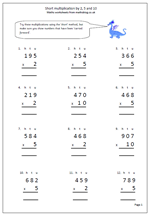# Short multiplication by 2, 5 and 10.When children are learning the standard methods of multiplication they need plenty of practice. With multiplication there are two distinct methods, the long and the short! Most schools tend to teach the long method first as it is clearer to show exactly what is happening, but it is also important for children to learn the short method, as we are looking to achieve fast accurate calculating.

This page keeps it simple by just multiplying by 2, 5 and 10; three tables that children should know ‘off by heart’.The questions have been laid out in the correct way so all that has to be done is:

a. Firstly to multiply by the units, remembering to carry any tens across to the tens column. These are usually placed under the answer.

b. Secondly multiply the tens remembering to carry any hundreds across to the hundreds column

c. Finally multiply the hundreds and write in the answer, including any thousands.

Short multiplication by 2, 5 and 10